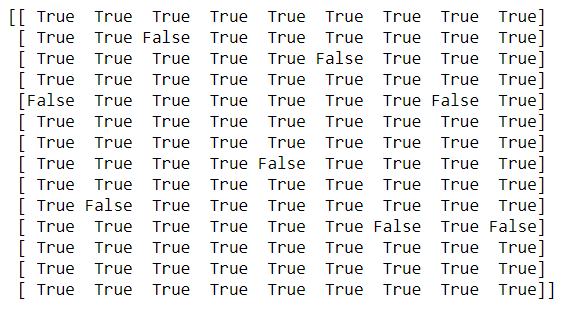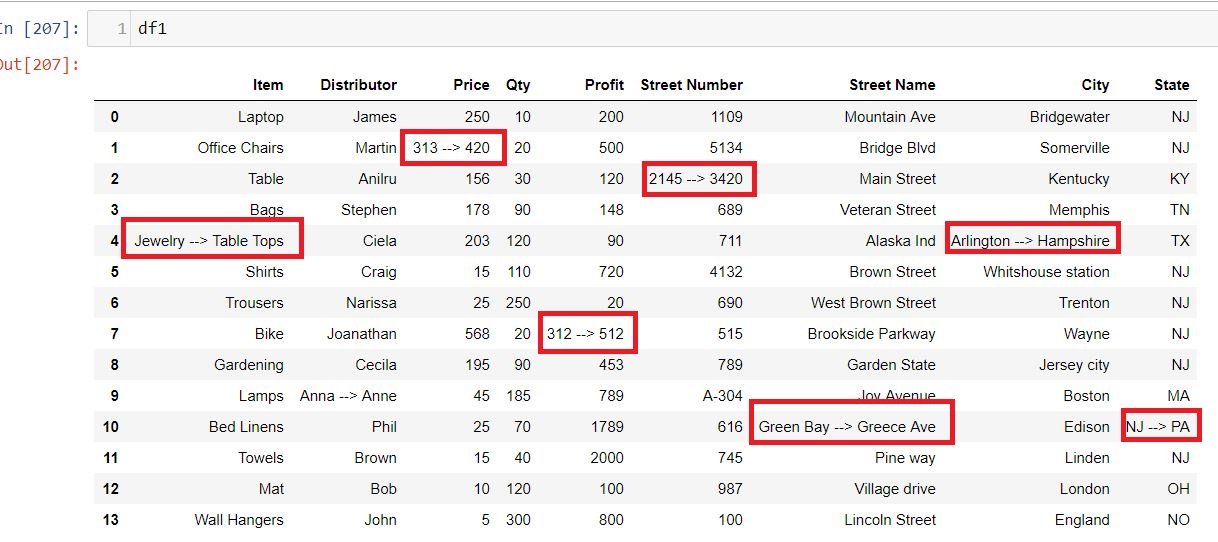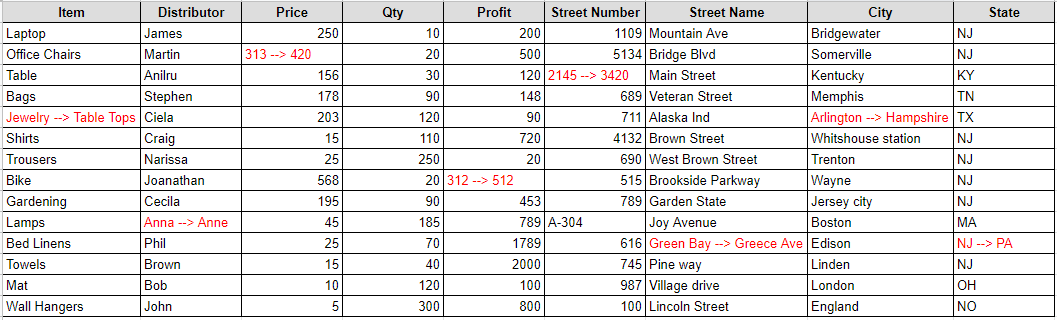# Compare two excel files for difference using Python

Comparing two excel spreadsheets and writing difference to a new excel was always a tedious task and Long Ago, I was doing the same thing and the objective there was to compare the row,column values for both the excel and write the comparison to a new excel files. In those days I have used xlrd module to read and write the comparison result of both the files in an excel file. I can still recall that we have written long lines of code to achieve that.

Recently at work, I encountered the same issue and retrieving my old xlrd script was not an option. So, i thought to give Pandas a try and amazingly I completed comparing the two excel files and writing the results to a new excel file in not more than 10 line of codes. I’m pretty sure that if I spend some more time then I can optimize the code further but this was a quick code that I wrote almost in no time for comparing over 100K records in both the excel file.

Let’s Start

I was comparing two excel files which contains the sales record of all the assets which the company sells to their customers in EU/EMEA/NA/APAC region. The two excel files I’m using is sample records from two Months i.e. Jan and Feb 2019 and contains the same no. of rows and columns

Import

First we need to import the two excel files in two separate dataframes

``````import pandas as pd

``````

Next Step

Compare the No. of Columns and their types between the two excel files and whether number of rows are equal or not.

First,We will Check whether the two dataframes are equal or not using pandas.dataframe.equals , This function allows two Series or DataFrames to be compared against each other to see if they have the same shape and elements. NaNs in the same location are considered equal. The column headers do not need to have the same type, but the elements within the columns must be the same dtype

This function requires that the elements have the same dtype as their respective elements in the other Series or DataFrame

Basically, it checks for the following three things between two dataframe:

a) They have the same types and values for their elements and column labels b) They have the same element types and values, but have different types for the column labels c) They have different types for the same values for their elements

``````df1.equals(df2)
``````

Compare Two Dataframe Values

In the above step we ensure that the shape and type of both the dataframes are equal and now we will compare the values of two dataframes

``````comparison_values = df1.values == df2.values
print (comparison_values)
``````

In just one line we have compared the values of two dataframes and the comparison value for each row and column is shown as True and False valuesIndex of the Cell with False value

Get the Index of all the cells where the value is False, Which means the value of the cell differ between the two dataframes.

``````import numpy as np
rows,cols=np.where(comparison_values==False)
``````

Next we will iterate over these cells and update the first dataframe(df1) value to display the changed value in second dataframe(df2)

``````for item in zip(rows,cols):
df1.iloc[item, item] = '{} --> {}'.format(df1.iloc[item, item],df2.iloc[item, item])
``````

Export to Excel

Finally we have replaced the old value of dataframe(df1) and entered the new value in the following format:

dfl (Old Value) —-> df2(New Value)

Here is how the updated dataframe(df1) looks like:So wherever there was a false value in the Comparison_value ndarray in the above step that has been replaced with the old and new value. Now you can export this dataframe into an excel or csv file and name it as Excel_diff.

I have set the index parameter as false otherwise the index will also be exported in the xlsx file as the first column and I have set the headers as True so that by default the dataframe headers will be the header in excel file as well.

``````df1.to_excel('./Excel_diff.xlsx',index=False,header=True)
``````Conclusion

Now if I compare my yesteryear code with the new and fast Pandas code then it really amuse me that how fast we have progressed and with the advent of modules like Pandas the things have become much simpler. Even you can directly read the records from SQL tables and write to the tables after processing. This new world is progressing at a faster speed and we all are optimistic with every day goes by we are near to see more intelligent and breakthroughs in the Python world.

Tags:

Categories:

Updated: